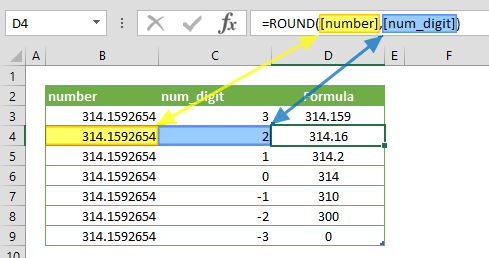The ROUND function allows you to manage the decimal portion of a numeric value. It rounds a number to a specified number of digits. In this guide, we’re going to show you how to use the ROUND function and also throw in some tips and error handling methods.

• All versions

# Syntax

ROUND(number,[num_digits])

# Arguments

 number The number to be rounded. [num_digits] The number of digits.

Examples

Firstly a reminder: Named ranges are added to ease of reading formulas.

## Example 1

=ROUND(314.159265358979,2)
formula returns 314.16; because when num_digits is greater than 0, then number is rounded to the specified number of decimal places.## Example 2

=ROUND(314.159265358979,0)
formula returns 314; because when num_digits is equal to 0, then number is rounded to the nearest integer.## Example 3

=ROUND(314.159265358979,-2)
formula returns 300; because when num_digits is less than 0, the number is rounded to the left of the decimal point.# Tips

• The ROUND function rounds number 1 to 4 down and 5 to 9 up.
• Use the ROUNDUP to always round up.
• Use the ROUNDDOWN to always round down.